Test: Regular Expressions & Languages- 1

# Test: Regular Expressions & Languages- 1

Test Description

## 20 Questions MCQ Test GATE Computer Science Engineering(CSE) 2023 Mock Test Series | Test: Regular Expressions & Languages- 1

Test: Regular Expressions & Languages- 1 for Computer Science Engineering (CSE) 2023 is part of GATE Computer Science Engineering(CSE) 2023 Mock Test Series preparation. The Test: Regular Expressions & Languages- 1 questions and answers have been prepared according to the Computer Science Engineering (CSE) exam syllabus.The Test: Regular Expressions & Languages- 1 MCQs are made for Computer Science Engineering (CSE) 2023 Exam. Find important definitions, questions, notes, meanings, examples, exercises, MCQs and online tests for Test: Regular Expressions & Languages- 1 below.
Solutions of Test: Regular Expressions & Languages- 1 questions in English are available as part of our GATE Computer Science Engineering(CSE) 2023 Mock Test Series for Computer Science Engineering (CSE) & Test: Regular Expressions & Languages- 1 solutions in Hindi for GATE Computer Science Engineering(CSE) 2023 Mock Test Series course. Download more important topics, notes, lectures and mock test series for Computer Science Engineering (CSE) Exam by signing up for free. Attempt Test: Regular Expressions & Languages- 1 | 20 questions in 60 minutes | Mock test for Computer Science Engineering (CSE) preparation | Free important questions MCQ to study GATE Computer Science Engineering(CSE) 2023 Mock Test Series for Computer Science Engineering (CSE) Exam | Download free PDF with solutions
 1 Crore+ students have signed up on EduRev. Have you?
Test: Regular Expressions & Languages- 1 - Question 1

### Which one of the following regular expressions represents the language: the set of all binary strings having two consecutive 0's and two consecutive 1's?

Detailed Solution for Test: Regular Expressions & Languages- 1 - Question 1

Set of all binary strings having two consecutive 0s and two consecutive1s

Anything 00 Anything 11 Anything + Anything 11 Anything 00 Anything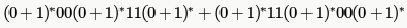And it is same after taking common.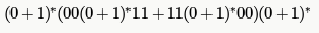Neither they said Both are immediate nor they give a predefined order, so it should be as above

*Multiple options can be correct
Test: Regular Expressions & Languages- 1 - Question 2

### Choose the correct alternatives (more than one may be correct) and write the corresponding letters only. Let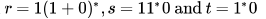be three regular expressions. Which one of the following is true?

Detailed Solution for Test: Regular Expressions & Languages- 1 - Question 2

to know the ans let us check all the options.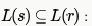strings generated by S are any numbers of 1's followed by one 0, i.e., 10,110,1110,1110,......) Strings generated by r are is 1 followed by any combination of 0 or 1, i.e., 1,10,11,1110,101,110,.....This shows that all the strings that can be generated by S,can also be generated by r it means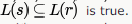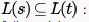here strings generated by t are any numbers of 1 (here 1* means we have strings as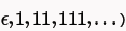followed by only one 0, i.e., 0,10,110,1110,.....So we can see that all the strings that are present in S can also be
generated by t, hence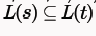which shows that option A is true.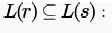this is false because string 1 which can be generated by r, cannot be generated by S

Same as option A.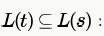. this is false because string 0 which can be generated by t ,cannot be generated by S .

Test: Regular Expressions & Languages- 1 - Question 3

### Choose the correct alternatives (more than one may be correct) and write the corresponding letters only: Which of the following regular expression identities is/are TRUE?

Detailed Solution for Test: Regular Expressions & Languages- 1 - Question 3

(b) RHS generates ∑* while LHS can't generate strings where r comes after s like sr,srr etc. LHS ⊂ RHS.

(c) LHS generates ∑* while RHS can't generate strings where r comes after an S. RHS  ⊂ LHS.

(d) LHS contains all strings where after an s, no r comes. RHS contains all strings of eitherr or s but no combination of them. So, RHS ⊂ LHS.

Test: Regular Expressions & Languages- 1 - Question 4

In some programming language, an identifier is permitted to be a letter followed by any number of letters or digits. If  and  denote the sets of letters and digits respectively, which of the following expressions defines an identifier?

Detailed Solution for Test: Regular Expressions & Languages- 1 - Question 4

It has to be started by a letter followed by any number of letters and digits.

Test: Regular Expressions & Languages- 1 - Question 5

Which two of the following four regular expressions are equivalent? (ε is the empty string).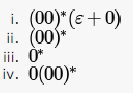Detailed Solution for Test: Regular Expressions & Languages- 1 - Question 5

you can have any no. of 0's as well as null.
A is false because you cannot have single 0 in ii). same for option B. In D you are forced to have single 0 in iv) whereas not in iii).

Test: Regular Expressions & Languages- 1 - Question 6

Which one of the following regular expressions over {0,1} denotes the set of all strings not containing  as substring?

Detailed Solution for Test: Regular Expressions & Languages- 1 - Question 6

"A regular expression denoting a language (set of strings) means it should generate all string in L and notgenerate any string not in L"

(a) - generates 100
(b) doesn't generate 0 (start trying strings in lexicographic order- 0, 1, 00, 01, 10,...)
(c) doesn't generate 1

Test: Regular Expressions & Languages- 1 - Question 7

If the regular set A is represented by A — (01 + 1)* and the regular set B is represented by B — ((01)*1*)*, which of the following is true?

Detailed Solution for Test: Regular Expressions & Languages- 1 - Question 7

Both generates all strings over {0,1} where a 0 is immediately followed by a 1.

*Multiple options can be correct
Test: Regular Expressions & Languages- 1 - Question 8

The string 1101 does not belong to the set represented by

Detailed Solution for Test: Regular Expressions & Languages- 1 - Question 8

Only (a) and (b) can generate 1101.

R.E of option C won’t generate 1101 as you can see the language will contain L(C) = {epsilon,10,1010,1001,0101,00,11,0011,1100,………..}
Also, R.E of option D has ‘1’ but here two ’11’ are together hence its impossible to generate 1101.L(D) = {Epsilon,0,00,110,11110,11000,…………….}

Test: Regular Expressions & Languages- 1 - Question 9

Lets' and T be languages over S = {a. b} represented by the regular expressions (a + b*)* and (a + b)*, respectively. Which of the following is true?

Detailed Solution for Test: Regular Expressions & Languages- 1 - Question 9

Both generates all strings over ∑ .

Test: Regular Expressions & Languages- 1 - Question 10

The regular expression 0*(10*)* denotes the same set as

Detailed Solution for Test: Regular Expressions & Languages- 1 - Question 10

(A) is the answer. Both (A) and the given expression generates all strings over ∑ .

(B) doesn't generate 11

(C) doesn't generate 11

Test: Regular Expressions & Languages- 1 - Question 11

Which of the following statements is TRUE about the regular expression 01*0?

Detailed Solution for Test: Regular Expressions & Languages- 1 - Question 11

Infinite set (because of *) of finite strings. A string is defined as a FINITE sequence of characters and hence can never be infinite.

Test: Regular Expressions & Languages- 1 - Question 12

Which one of the following regular expressions is NOT equivalent to the regular expression (a + b + c)*?

Detailed Solution for Test: Regular Expressions & Languages- 1 - Question 12

A) (a* + b* + c*)*  = ( ^ + a+aa+.. ..+b+bb+...+c+cc...)* = (a+b+c+ aa+..+bb+..+cc+..)*= (a+b+c)*  [any combination of rest of aa ,bb,cc, etc already come in (a+b+c)* ]

(a*b*c*)* = (a*+b*+c* +a*b*+b*c*+a*c*+..)*= (a+b+c+....)* = (a+b+c)*

((ab)* + c*)* =(ab+c+^+abab+...)* = (ab+c)*

(a*b* + c*)* = (a*+b*+c*+...)* =(a+b+c+..)* = (a+b+c)*

Test: Regular Expressions & Languages- 1 - Question 13

Which regular expression best describes the language accepted by the non-deterministic automaton below?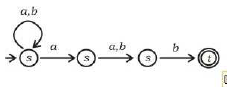Detailed Solution for Test: Regular Expressions & Languages- 1 - Question 13

Say s1, s2 , s3 and t are states (in sequence)

s1 is start state
s1= ^ + s1a + s1b = ^+s1(a+b) = (a+b)* [using arden's theorem  R= Q+RP , then R= QP*][^ bcoz of start state]
s2 = s1a = (a+b)*a

s3= s2a+s2b = s2(a+b) = (a+b)*a(a+b)

t= s3b= (a+b)*a(a+b)b

t is final state so regular expression is (a+b)*a(a+b)b

Test: Regular Expressions & Languages- 1 - Question 14

Consider the regular expression R = (a + b)* (aa + bb) (a + b)*

Which one of the regular expressions given below defines the same language as defined by the regular expression R?

Detailed Solution for Test: Regular Expressions & Languages- 1 - Question 14

R = (a+b)*(aa+bb)(a+b)*

having NFA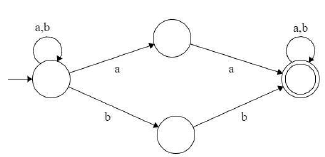Equivalent DFA :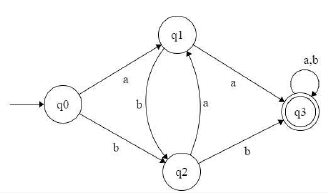which is equivalent Transition graph [ by removing transition from q1 to q2 and q2 to q1 but does not effect on language ..be careful ]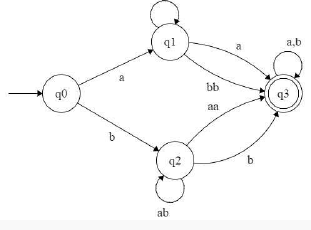That is equivalent to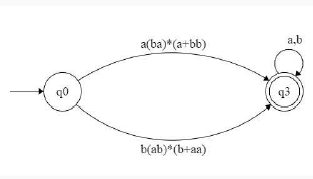which is equivalent to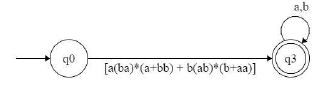which is equivalent to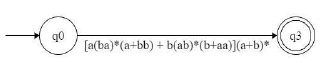so equivalent regular expression is [a(ba)*(a+bb) + b(ab)*(b+aa)](a+b)* so option C is answer.

Test: Regular Expressions & Languages- 1 - Question 15

Which of the following regular expressions describes the language over{0, 1} consisting of strings that contain exactly two 1's?

Detailed Solution for Test: Regular Expressions & Languages- 1 - Question 15

A) with at least 2 consecutive 1's, any no of 0's and any no of 1's

B) exactly two consecutive 1's

C)exactly two 1's but need not be consecutive

D) any no of 1's and 0's with at least two 1's

Test: Regular Expressions & Languages- 1 - Question 16

Which one of the following languages over the alphabet {0,1} is described by the regular expression: (0 + 1)*0(0 + 1)*0(0 + 1)*?

Detailed Solution for Test: Regular Expressions & Languages- 1 - Question 16

Counter example for other choices:

(A) 1010 is accepted which doesn't contain 00

(B) 000 is accepted

(D) 01 is not accepted

Test: Regular Expressions & Languages- 1 - Question 17

Let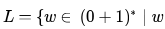has even number of 1s}  i.e., L is the set of all the bit strings with even numbers of 1s. Which one of the regular expressions below represents L?

Detailed Solution for Test: Regular Expressions & Languages- 1 - Question 17

(A) - If the string contains a 1, it must end in a 1 hence cannot generate all bit strings with even number of 1's (eg, 1010)
(C) - between the second and third 1's a 0 is not allowed (eg, 011011)

(D) - 00 is not allowed, zero is an even number.

Test: Regular Expressions & Languages- 1 - Question 18

Which of the regular expressions given below represent the following DFA?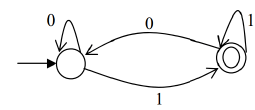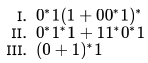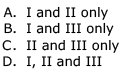Detailed Solution for Test: Regular Expressions & Languages- 1 - Question 18

(B) is the answer. (II) doesn't generate 11011 which is accepted by the given DFA

Test: Regular Expressions & Languages- 1 - Question 19

Let r,s,t be regular expressions. Which of the following identities is correct?

Detailed Solution for Test: Regular Expressions & Languages- 1 - Question 19

a. (r + s)* = r*s*            LHS can generate 'sr' but RHS not

b. r(s + t) = rs + t          LHS can generate 'rt' but RHS not

c. (r + s)* = r* + s*       LHS can generate 'sr' but RHS not

d. (rs + r)* r = r (sr + r)*   They are equivalent

e. (r*s)* = (rs)*            LHS can generate 'rrrs' but RHS not

Test: Regular Expressions & Languages- 1 - Question 20

Which of the following regular expressions correctly accepts the set of all 0/1-strings with an even (poossibly zero) numbe of 1s?

Detailed Solution for Test: Regular Expressions & Languages- 1 - Question 20

As, mentioned in the question, the regular expression must accept all strings of 0 and 1 but with even no of 1s (including no 1s). Hence, 00 must be in the language. Option a, b, c, d do not accept 00. Hence, option e is correct.

## GATE Computer Science Engineering(CSE) 2023 Mock Test Series

150 docs|215 tests
 Use Code STAYHOME200 and get INR 200 additional OFF Use Coupon Code
Information about Test: Regular Expressions & Languages- 1 Page
In this test you can find the Exam questions for Test: Regular Expressions & Languages- 1 solved & explained in the simplest way possible. Besides giving Questions and answers for Test: Regular Expressions & Languages- 1, EduRev gives you an ample number of Online tests for practice

## GATE Computer Science Engineering(CSE) 2023 Mock Test Series

150 docs|215 tests